# Chapter 13 Surface Areas and Volumes RS Aggarwal Solution for Class 9th Maths

0
2098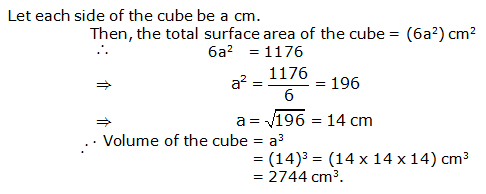Volume andSurface Area Exercise 13A Questio3n 1: 3 length =12cm, breadth = 8 cm and height = 4.5 cm ∴ Volume o2f cuboid = l x b x h = (12 x 28 x 4.5) cm2= 432 cm ∴ Lateral surface area of a cuboid = 2(l + b) x h = [2(12 + 8) x 4.5] cm 2 = (2 x 20 x2 4.5) cm = 180 cm ∴ T2otal surface area cuboid = 2(lb +b h+ l h) 2 = 2(12 x 8 + 8 x 4.5 + 12 x 4.5) cm = 2(96 +36 +54) cm = (2 x186) cm = 372 cm Length 236 m, breadth =14 m and height =6.5 m ∴ V3olume of a cuboid = l x b x h = (26 x 14 x 6.5) m = 2366 m 2 ∴ Lateral s2urface area of a cuboid =2 (l + b) x h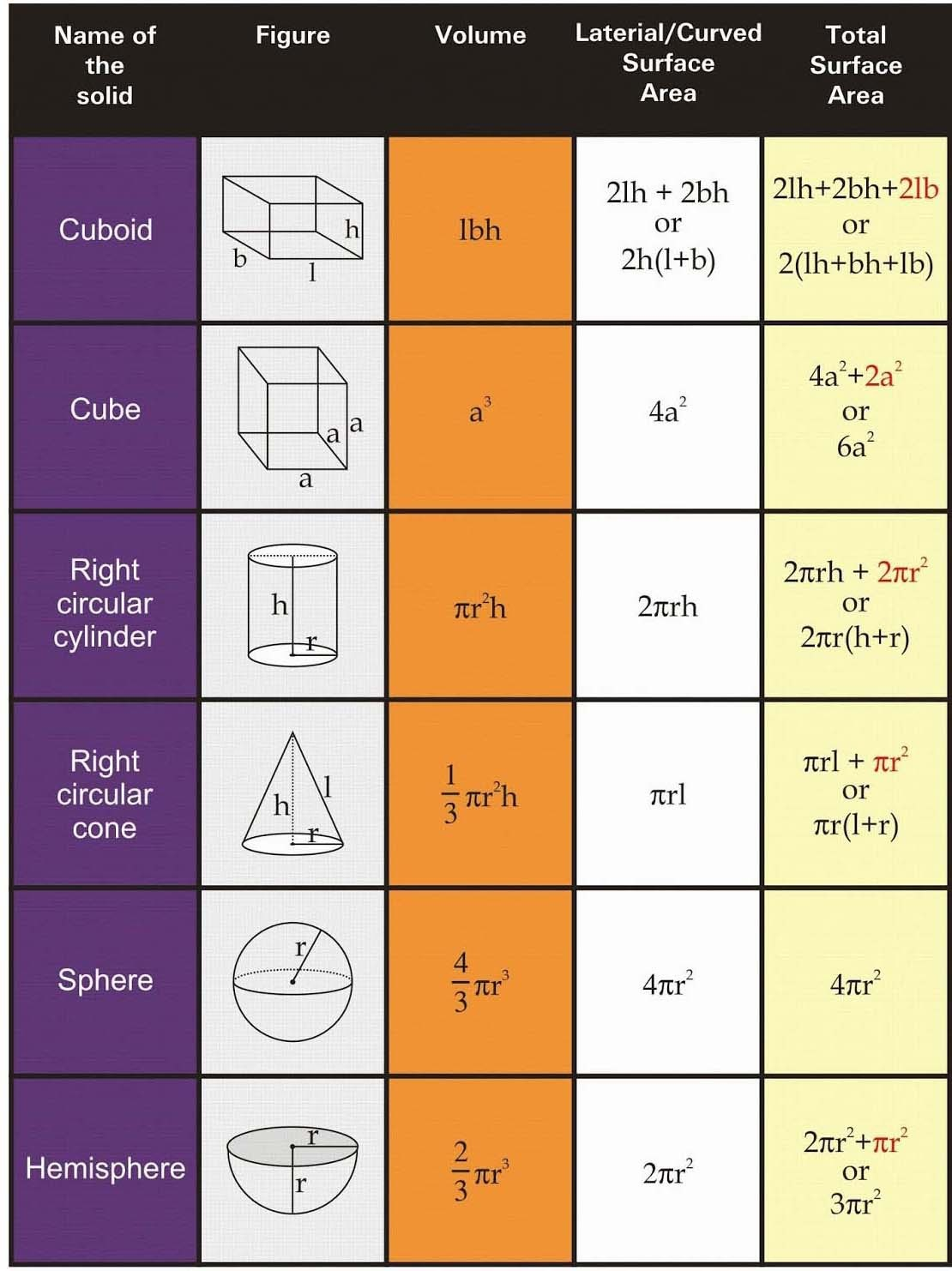= [2(26+14) x 6.5] m

= (2 x 40 x 6.5) m

= 520 m2

∴ Total surface area = 2(lb+ bh + lh)

= 2(26 x 14+14 x6.5 +26 x6.5)

= 2 (364+91+169) m2

= (2 x 624) m2= 1248 m2.

1. Length = 15 m, breadth = 6m and height = 5 dm = 0.5 m

∴ Volume of a cuboid = l x b x h

= (15 x 6 x 0.5) m3=45 m3.

∴ Lateral surface area = 2(l + b) x h

= [2(15 + 6) x 0.5] m2

= (2 x 21×0.5) m2=21 m2

∴ Total surface area =2(lb+ bh + lh)

= 2(15 x 6 +6 x 0.5+ 15 x 0.5) m2

= 2(90+3+7.5) m2

= (2 x 100.5) m2

=201 m2

1. Length = 24 m, breadth = 25 cm =0.25 m, height = 6m.

∴ Volume of cuboid = l x b x h

= (24 x 0.25 x 6) m3.

= 36 m3.

∴ Lateral surface area = 2(l + b) x h

= [2(24 +0.25) x 6] m2

= (2 x 24.25 x 6) m2

= 291 m2.

∴ Total surface area =2(lb+ bh + lh)

=2(24 x 0.25+0.25x 6 +24 x 6) m2

= 2(6+1.5+144) m2

= (2 x151.5) m2

=303 m2.

Question 2:

Length of Cistern = 8 m Breadth of Cistern = 6 m

And Height (depth) of Cistern =2.5 m

∴ Capacity of the Cistern = Volume of cistern

∴ Volume of Cistern = (l x b x h)

= (8 x 6 x2.5) m3

=120 m3

Area of the iron sheet required = Total surface area of the cistem.

∴ Total surface area = 2(lb +bh +lh)

= 2(8 x 6 + 6×2.5+ 2.5×8) m2

= 2(48 + 15 + 20) m2

= (2 x 83) m2=166 m2

Question 3:

Length of a room =9m, Breadth of a room = 8m And height of room = 6.5 m

∴ Area of 4 walls = Lateral surface area

= 2 (l+ b) x h

= [2 (9+8) x 6.5] m2

= (2 x 17 x 6.5) m2

=221 m2

∴ Area not be whitewashed = (area of 1 door) + (area of 2 windows)

= (2 x 1.5) m2 + (2 x 1.5 x 1) m2

= 3m2 + 3m2 =6m2

∴ Area to be whitewashed = (221-6) m2 =215 m2

∴ Cost of whitewashing the walls at the rate of Rs.6.40 per Square meter = Rs. (6.40 x 215) = Rs. 1376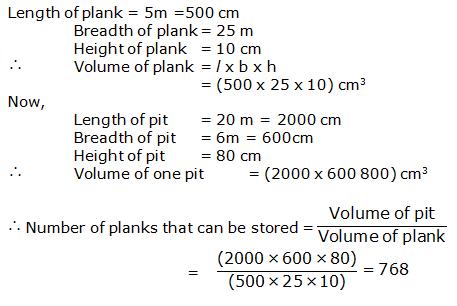Question 4: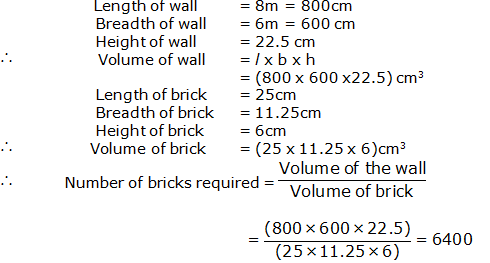Question 5: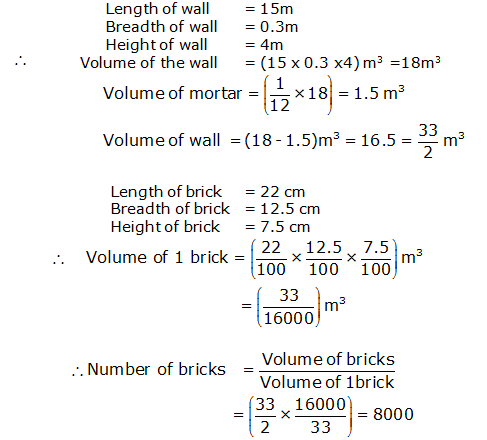Question 6: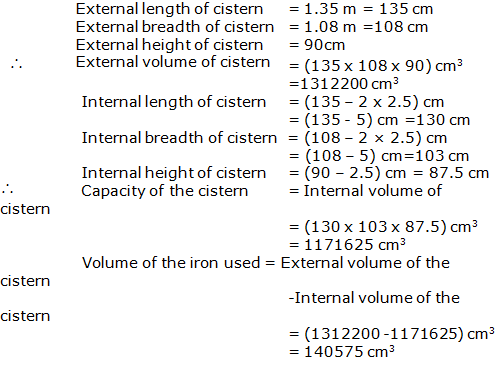Question 7:

Question 8: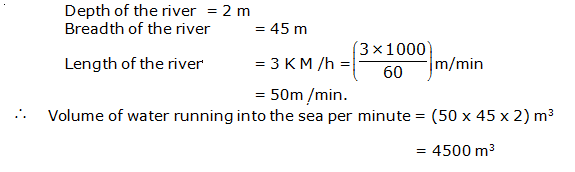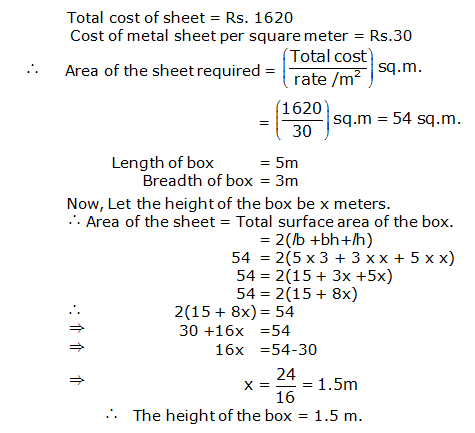Question 9: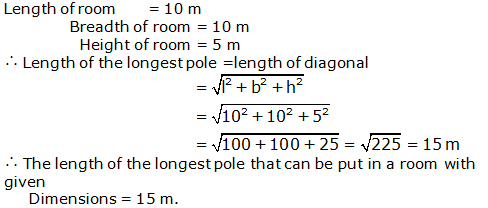Question 10: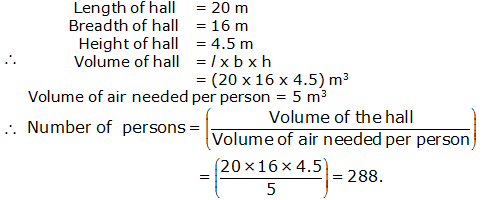Question 11:

Question 12: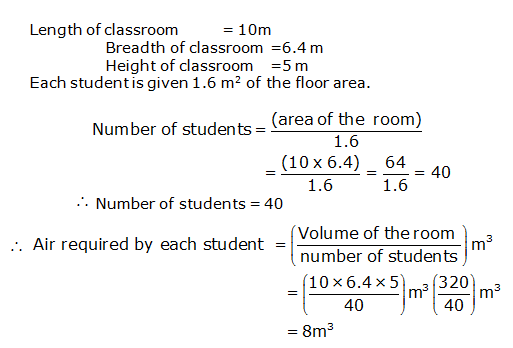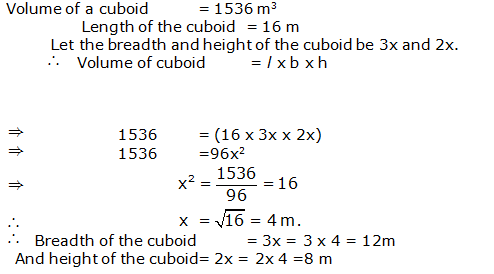Question 13: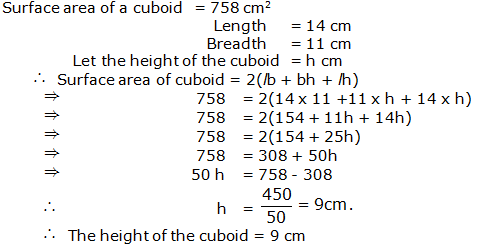Question 14:

Question 15: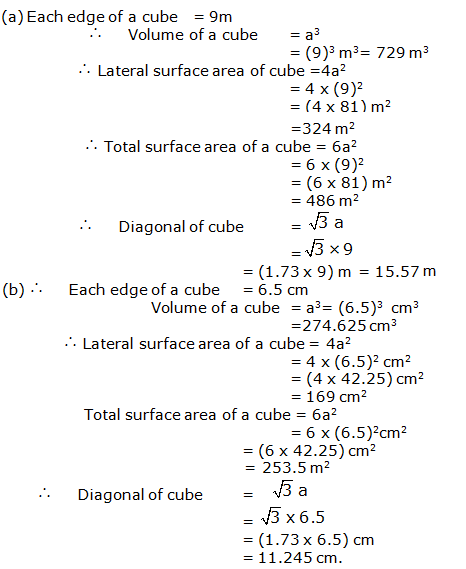Question 16: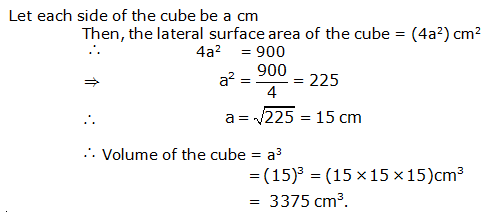Question 17: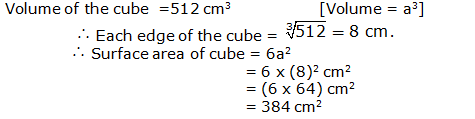Question 18:

Question 19: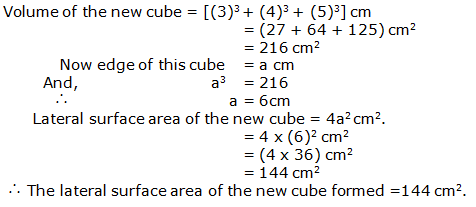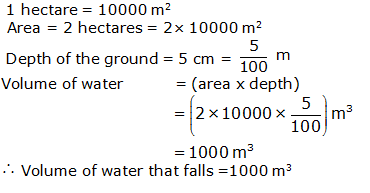Question 20:

Exercise 13B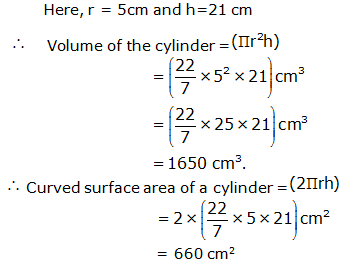Question 1:

Question 2: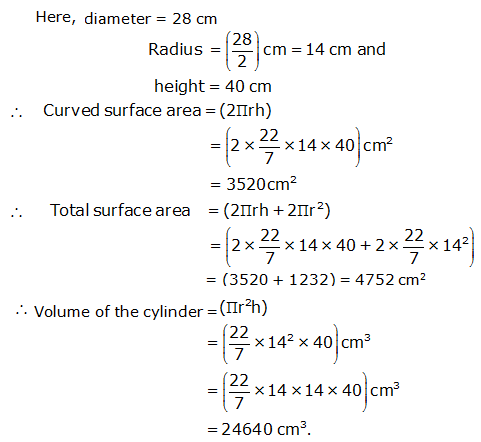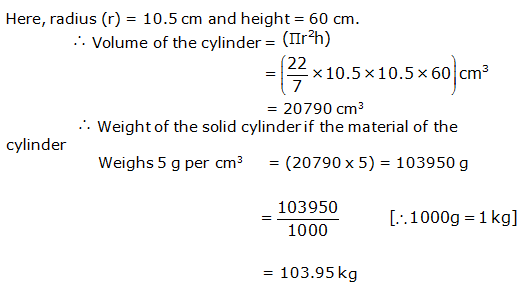Question 3: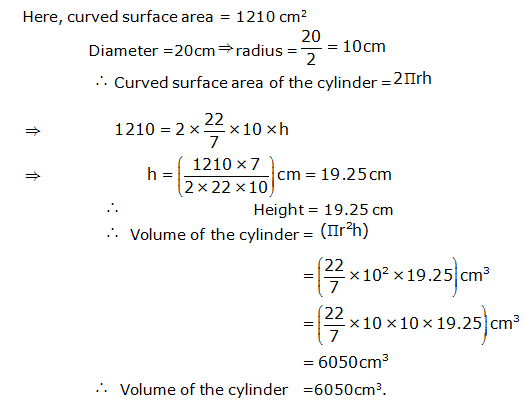Question 4:

Question 5: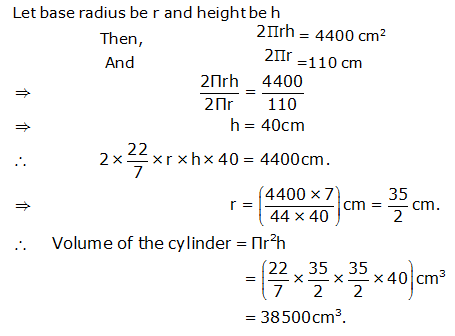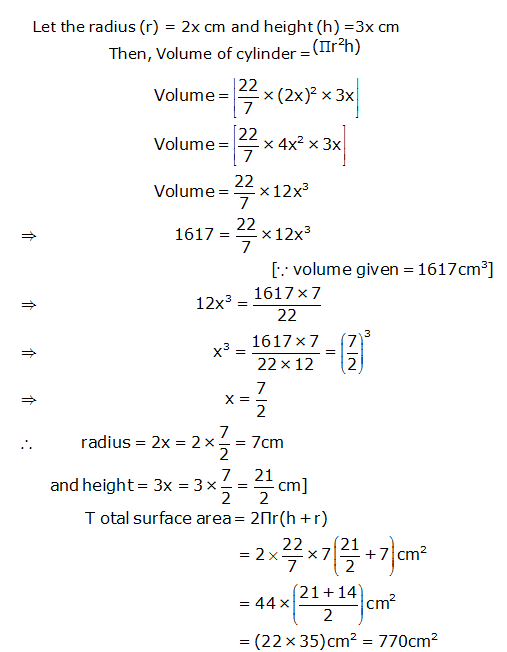Question 6:

Question 7: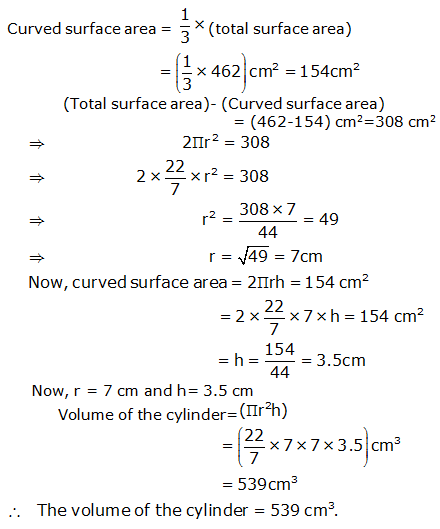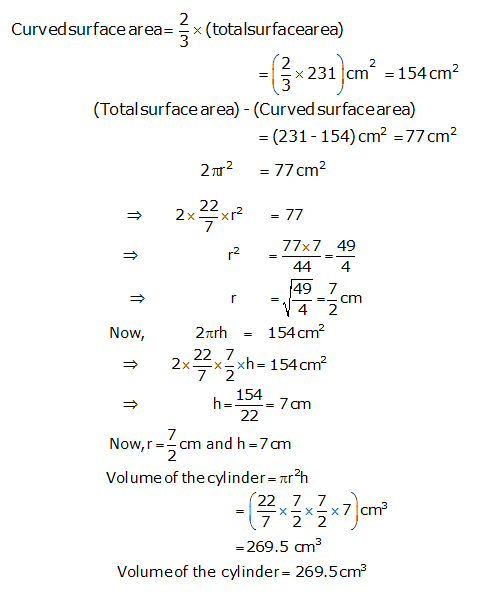Question 8:

Question 9: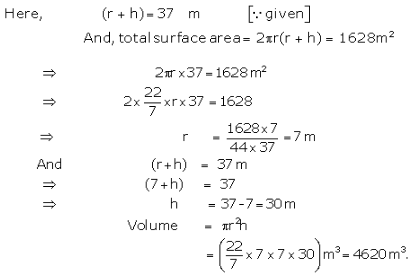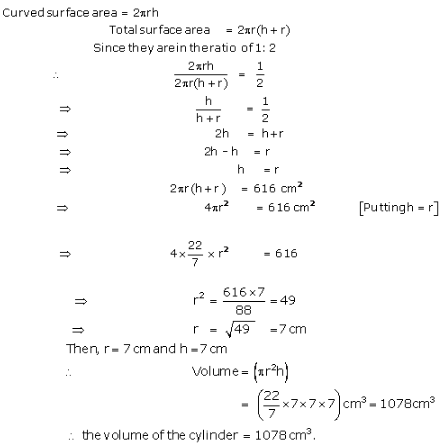Question 10: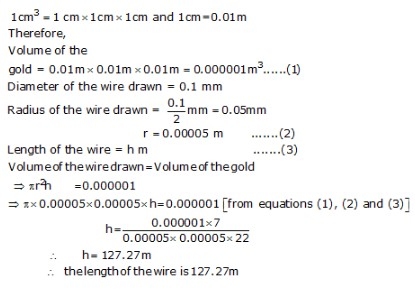Question 11:

Question 12: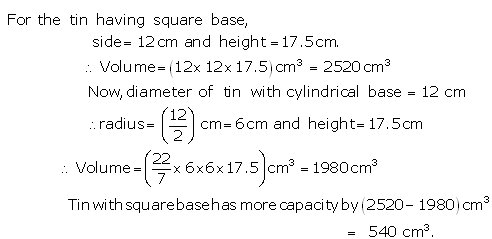Question 13: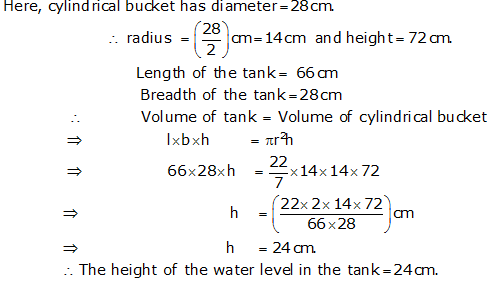Question 14:Question 15:

Question 16: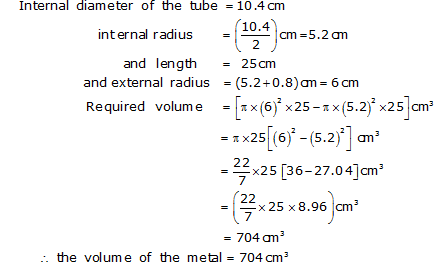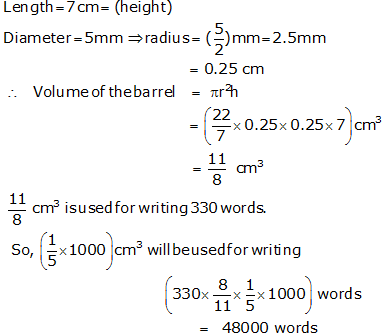Question 17: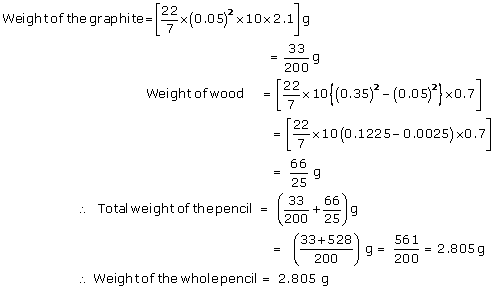Question 18:

Question 1:

Exercise 13C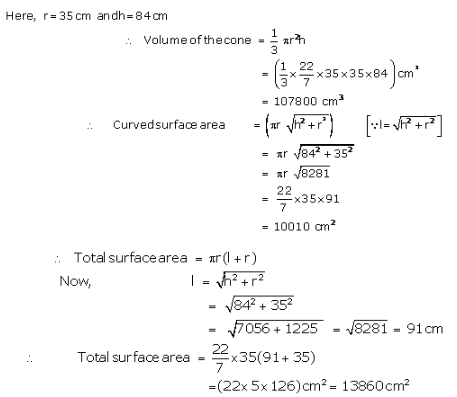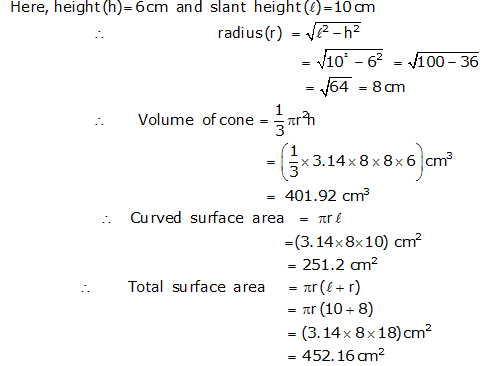Question 2: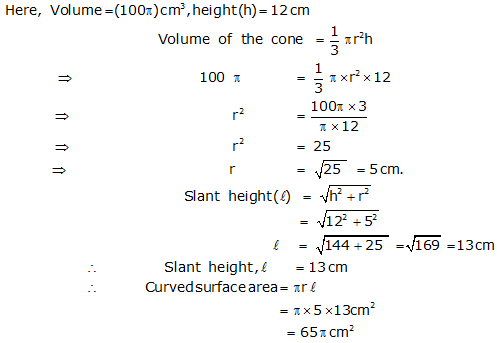Question 3:

Question 5: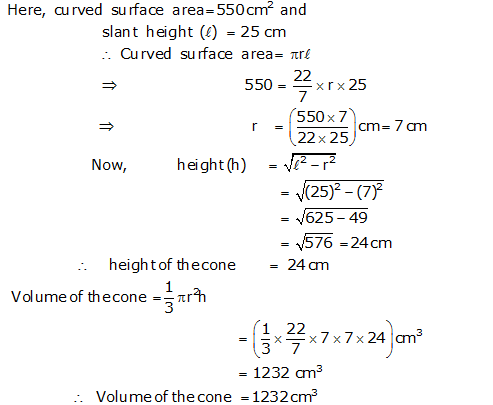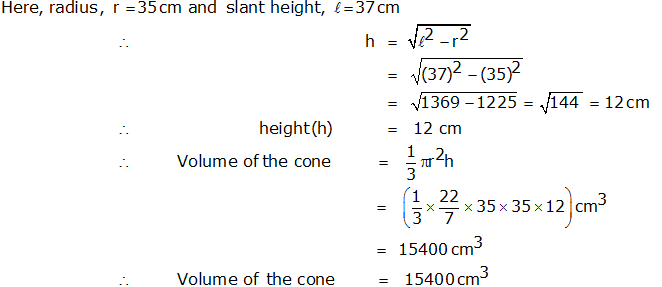Question 6: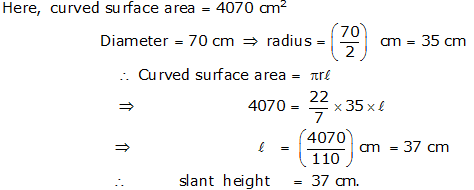Question 7: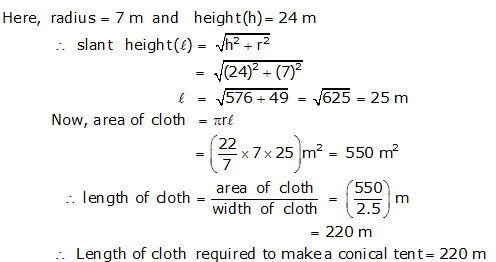Question 8:

Question 9: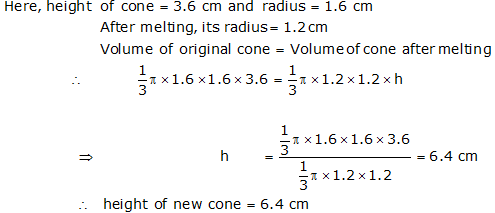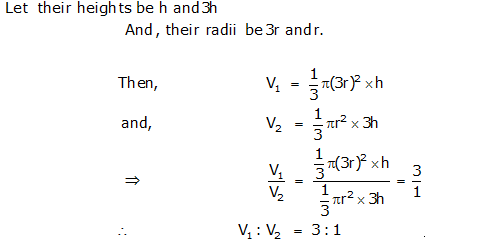Question 10: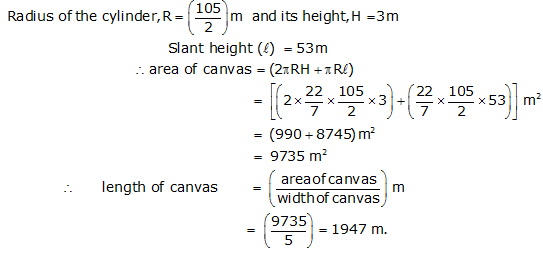Question 11: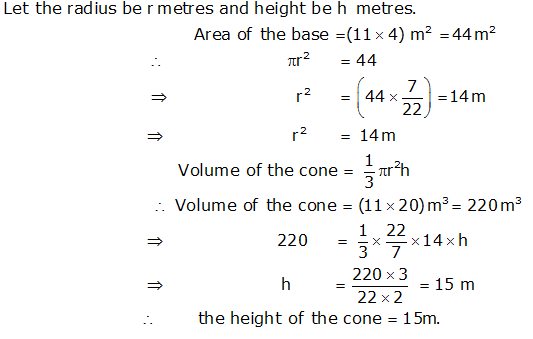Question 12:

Question 13: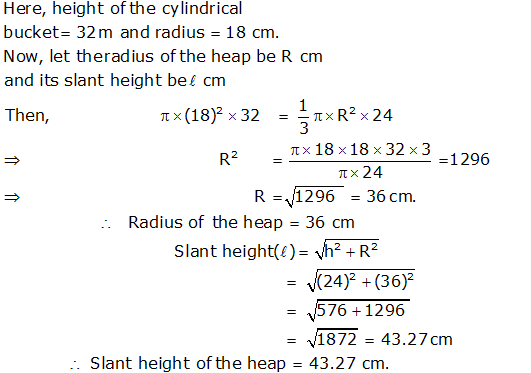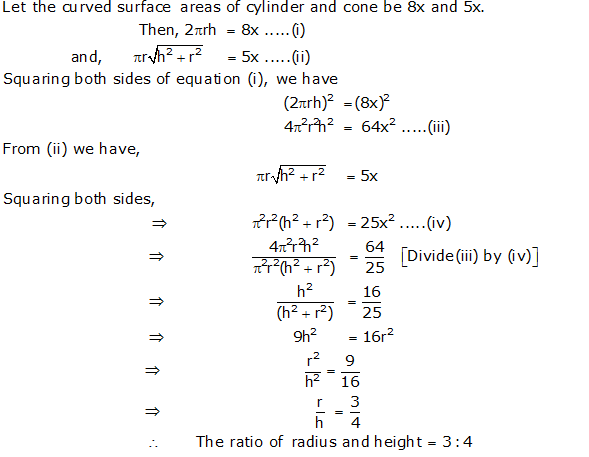Question 14: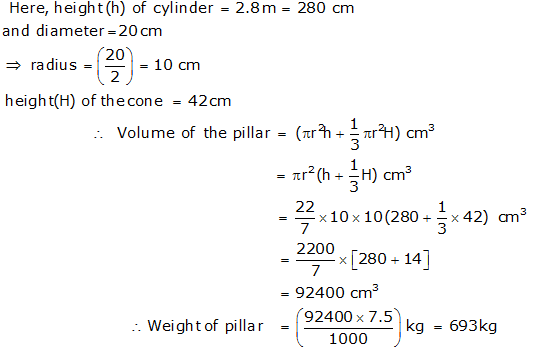Question 15:

Question 16: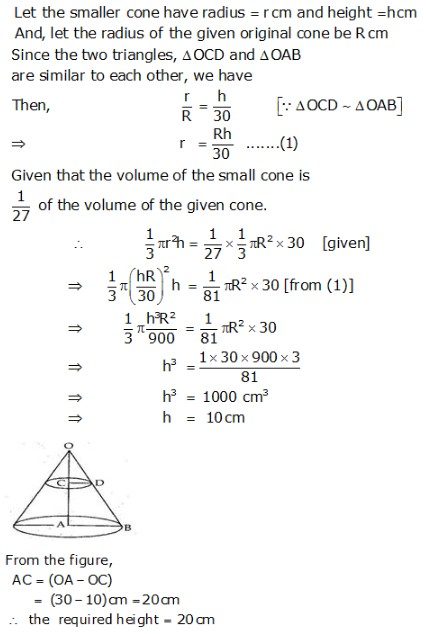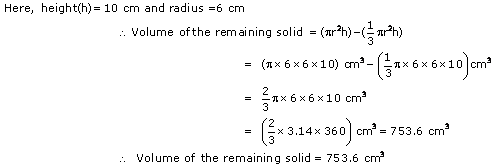Question 17: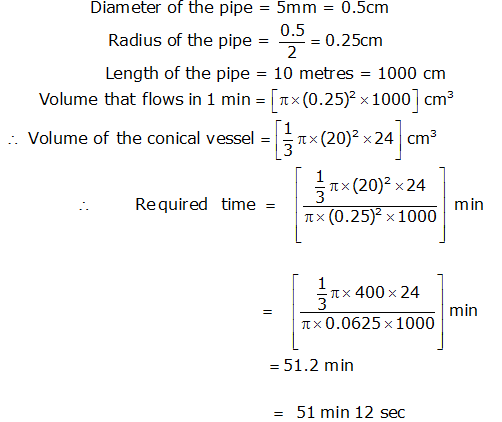Question 18:

Exercise 13D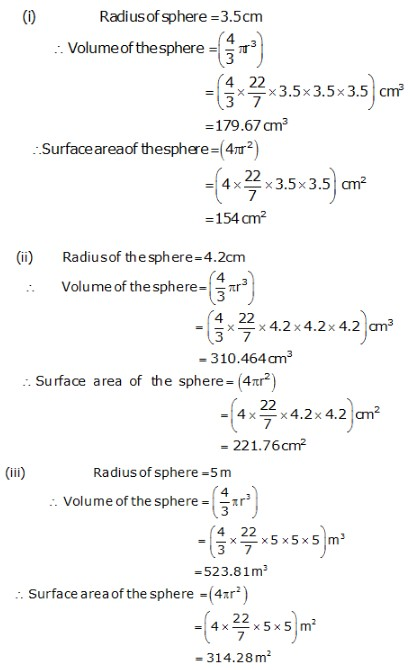Question 1:

Question 2: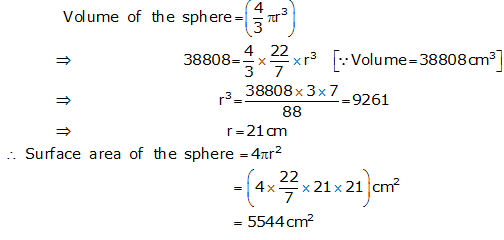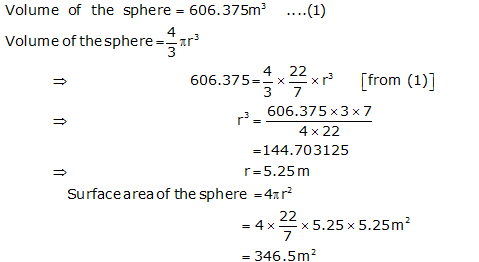Question 3: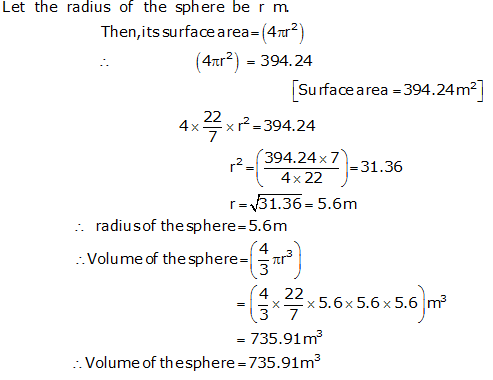Question 4:

Question 5: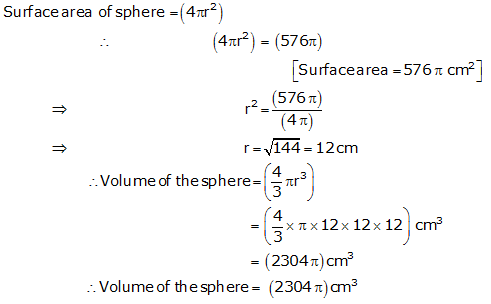Question 6: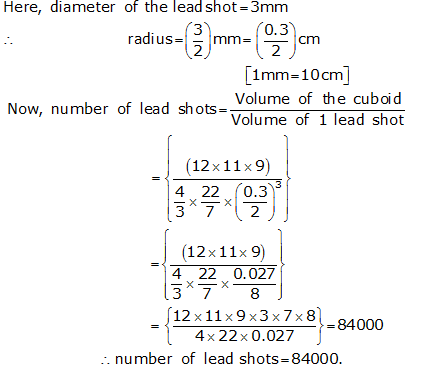Question 7: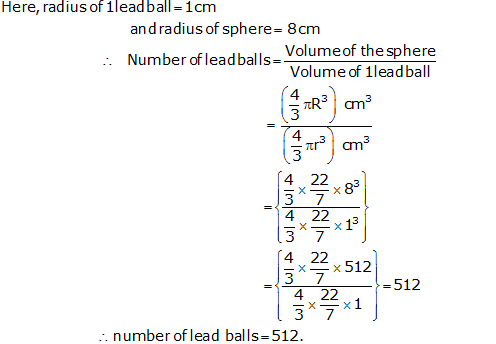Question 8: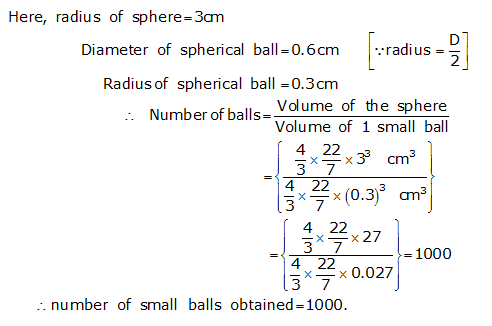Question 9: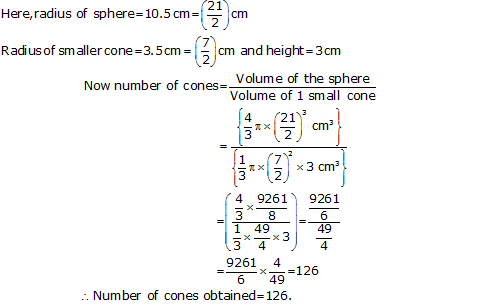Question 10:

Question 11: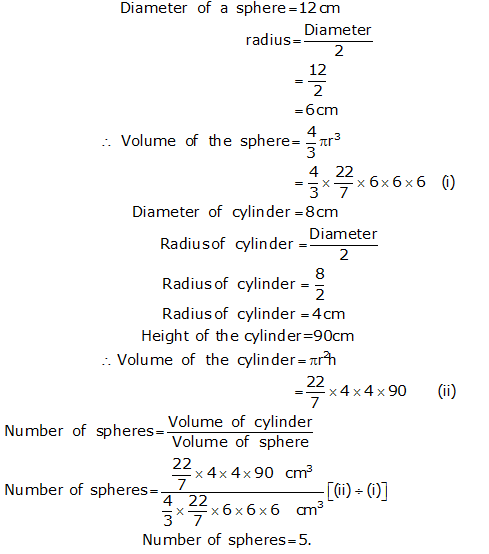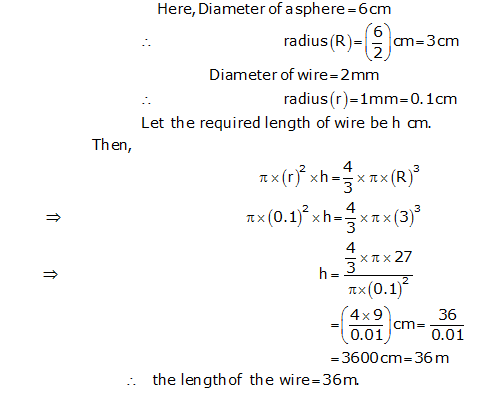Question 12:

Question 13: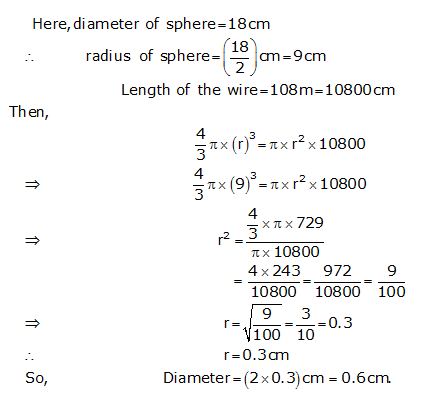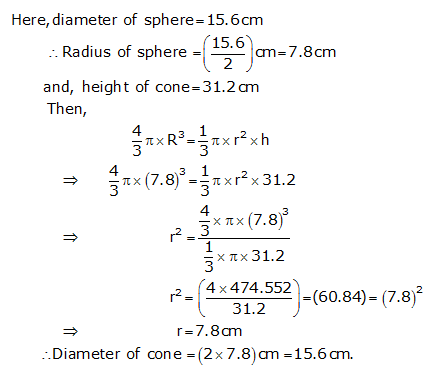Question 14: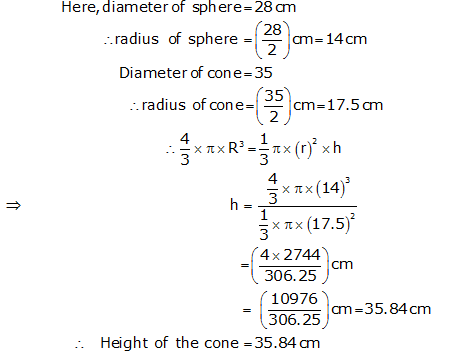Question 15:

Question 16: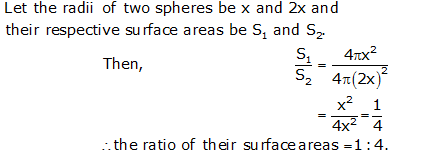Question 17: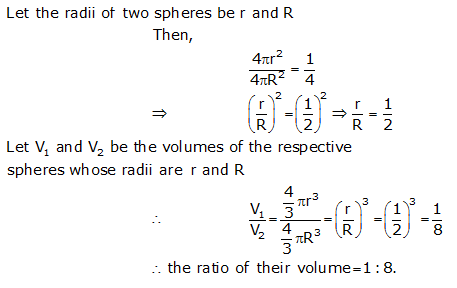Question 18:

Question 19: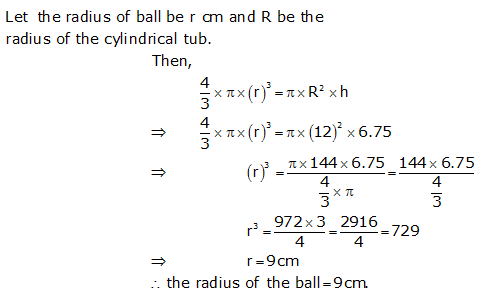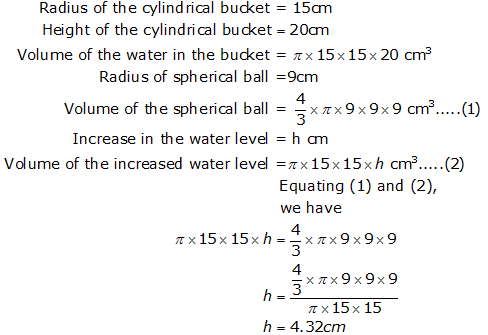Question 20:Question 21:

Question 22: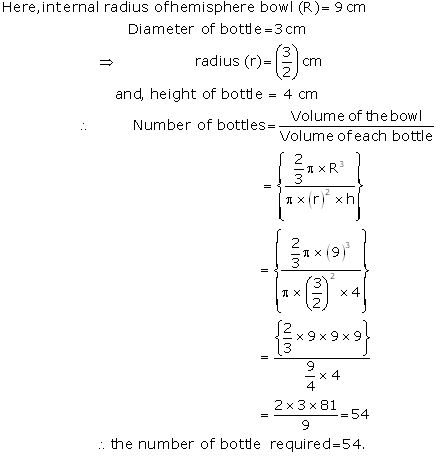Question 23:

Previous articleChapter 10 Circles RS Aggarwal Solution for Class 9th Maths
Next articleClass 9th Math R.D. Sharma Solutions